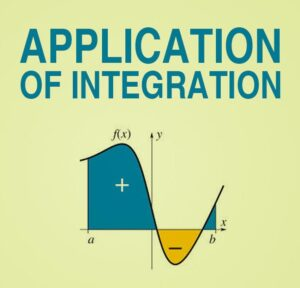Integration Applications JEE

# Integration Applications JEEInfinity Learn NEET Festival
Infinity Learn IIT JEE Festival

##Important Integral Application Questions in JEE Advanced
Calculations are made using a variety of approaches. Functions, differentiation, and integration are a few examples. The usage and application of integrals is one of them, and it is not restricted to the subject of mathematics. The usage of integrals can also be seen in scientific and engineering topics. This is due to the fact that integral formulae must be used to compute the area.

This page seeks to provide applicants with pertinent questions that will assist them in preparing for their IIT Examinations. The website assists and accompanies students through every stage of the preparation process, ensuring that they do not fall behind on anything.

Need FREE NCERT/CBSE/IIT-JEE/NEET Study Material?

## Integrals in Practice Important JEE Advanced Questions

Integrals in Practice When it comes to the JEE Advanced syllabus, Calculus is an important part of the Mathematics section. In the Joint Entrance Question Paper, roughly 40% of the questions come from this section. One of the most important chapters in Calculus is Integrals. Integrals will very certainly appear in four questions in the exam. For your convenience, the subtopics of the Integral unit are listed below.

 S. no. Sub-Topics 1 Indefinite integral 2 Definite integral 3 Integration using partial fractions 4 Integration by parts 5 properties of definite integral

## Importance of application of Integrals Chapter important for JEE

Yes, the topic of Engineering Application is crucial not only for the JEE advanced tests but also for those students who intend to pursue engineering as a bachelor’s degree. Candidates should expect 3-4 questions directly related to this topic. This chapter is estimated to be worth about 12 points. It is self-evident that each question has a significant impact on the candidates’ grades. As a result, in order to answer questions from this chapter, the student will need to prepare thoroughly and effectively. The applicant will only be able to answer the questions without losing marks if this is the case.

## FAQs

##### What is the definition of an integral?

A derivative function is referred to as an integral. Its primary function is to determine the area covered by two-dimensional regions. It may also be used to calculate the volumes occupied by three-dimensional objects. As a result, it's important to remember that determining an integral function of x entails calculating the area of the X axis from the curve. The antiderivative is another name for an integral. This is due to the fact that it reverses the differentiation process.

Q. What are some examples of integral applications?

Ans . Integrals are useful not just in mathematics, but also in physics. Its mathematical application is as follows:

1. It’s utilized to figure out where the centre of mass is in a curved region.

2. The integrals are also useful for calculating the area between two curves.

3. Integrals may also be used to calculate the area beneath a curve.

4. Knowing integrals will assist you in determining the average value of a curve.

Q. What are the different sorts of integrals?

Ans. Integrals are divided into two categories.

DEFINITE INTEGRALS: the definite integrals are defined as an integral comprising definite bounds, upper limit and lower limit. Riemann integrals are another name for them.

INDEFINITE INTEGRALS: the indefinite integrals are those that don’t have specified upper and lower boundaries.

Join Infinity Learn Regular Class Programme!